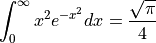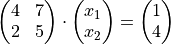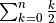# Edit=>Insert LaTeX Formula¶

(TeX is a typesetting system designed and mostly written by Donald E. Knuth.)

This command inserts LaTeX-formulas into the active document page.

A formula is typed in text form as follows:

$$\int_{0}^{\infty} x^2 e^{-x^2} dx = \frac{\sqrt{\pi}}{4}$$


The created formula will be inserted into the document page as a high resolution bitmap (png) with 600 dpi.To modify the formula, double-click the bitmap with the mouse.

More documtation about Latex can be found here http://www.latex-project.org/.

## Installation¶

Check if latex.exe and dvipng.exe are installed on your computer or if they are available on your network:

c:\> dvipng -version

dvipng 1.9
kpathsea version 3.3.2
There is NO warranty.  You may redistribute this software
under the terms of the GNU General Public License.
named COPYING and dvipng.c.

c:\> latex -version

MiKTeX-pdfTeX 2.7.3235 (1.40.9) (MiKTeX 2.7)
Copyright (C) 1982 D. E. Knuth, (C) 1996-2006 Han The Thanh
TeX is a trademark of the American Mathematical Society.


If Latex is not installed it is recommended to in install the free Latex distribution MiKTeX (http://www.miktex.org) from Christian Schenk. The setup program can be find here: http://www.miktex.org/2.9/setup. dvipng.exe is included.

There also a portable edition of MikTeX available (http://miktex.org/portable/about). It can be used from a USB stick without administrator privilieges. The path to the installation can be set in the UniPlot command window View=>Command Window). The following commands sets path, where j: is the drive letter of your USB stick:

* WriteProfileString("Settings", "latex-path", "j:\\miktex\\bin");


Check the set-up:

* _latex_find()
j:\miktex\bin\latex.exe
j:\miktex\bin\dvipng.exe


UniPlot saves temporary files in the user directory of UniPlot. If the path name contains spaces it may cause problems with some TeX distributions. In this case you can specify the name of new existing directory without spaces with the following command:

* WriteProfileString("settings", "latex_temp_path", "c:/temp");


## Example¶

\begin{pmatrix}
4 & 7 \\
2 & 5
\end{pmatrix}
\cdot
\begin{pmatrix}
x_{1} \\
x_{2}
\end{pmatrix}
=
\begin{pmatrix}
1 \\
4
\end{pmatrix}You can also write normal text with Latex and include a formula
like the following $\sum^{n}_{k=0}\frac{k}{2}$ into the text.


You can also write normal text with Latex and include a formula like the followinginto the text.